# Inelastic Collision

## Inelastic Collisions

• Inelastic collision is a collision where the kinetic energy is not conserved due to internal friction
• Though the kinetic energy is lost in these collision,but they obey law of conservation of Linear Momentum
• If the bodies or particles stick together and move together after the collision, the it is called Perfectly inelastic collision
• If the bodies or particles does stick together after the collision but there is loss of kinetic energy, the it is inelastic collision
• Coefficient of restitution for the inelastic collision lies as $0 \leq e < 1$. e is 0 for perfectly inelastic collision and lies between 0 and 2 for elastic collision
• Inelastic collision can be further divided into head on collision (i.e collision in one dimension) and opaque collision (i.e collision in two dimension)
• Lets study about these two inelastic collision in one dimension and two dimension further

## Head on inelastic collision of two particles which stick together

• Consider two particles whose masses are m1 and m2 .Let u1 and u2 be the respective velocities before collision
• Let both the particles stick together after collision and moves with the same velocity v .Then from law of conservation of linear momentum
m1u1 +m2u2=(m1+m2)v
or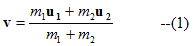• If we consider second particle to be stationary or at rest then u2=0
then
m1u1 =(m1+m2)
or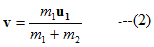Hence |v| < |u1|

• Kinetic energy before collision is
KE1=(1/2)m1u12

After collison KE of the system is
KE2=(1/2)(m1+m2)v2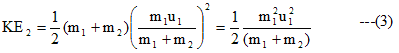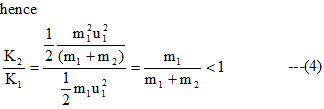Hence from equation we come to know that K2 < K1 hence energy loss would be there after thye collision of the particles

## (15) Deflection of an moving particle by a particle at rest ( inelastic collision in two dimension)

• Let m1 and m2 be the two mass particle in a laboratory frame of refrence and m1 collide with m2 which is initailly is at rest.Let the velocity of mass m1 before collison be u1 and after the collison it moves with a velocity v1 and is delfected by the angle θ1 withs its incident direction and
m2 after the collision moves with the velocity v2 and it is deflected by an angle θ2 with its incident direction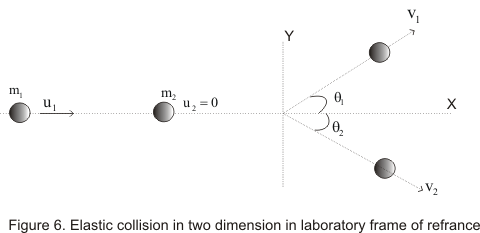• From law of conservation of linear momentum ,for components along x-axis
m1u1=m1v1cosθ1 +m2v2cosθ2 ---(1)
For components along y-axis
0=m1v1sinθ1 -m2v2sinθ2 --(2)

• Analysing above equations we come to know that we have to find values of four unknown quantities v1,v212 with the help of above three equations which is impossible as we need to have atleast four equations for finding out the values of four unknown quantities .So we cannot predict the variable as they are four of them . However if we measure any two variable ,then other variable can be uniquely determined from the above equation

Example-1
A 10 kg object is moving in +x direction at 30 m/s. An another object of mass 40 g is moving in -x direction at 50 m/s. They collide head on and stick together. Find the final velocity ?
Solution
This is a case of Perfectly inelastic collision, applying law of conservation of momentum
Momentum before impact = Momentum After Impact
$(10) \times 30 + (.04) \times (-50) = (10.04)v$
Negative sign is for 40g particle as it is moving in negative direction
v=29.68 m/s